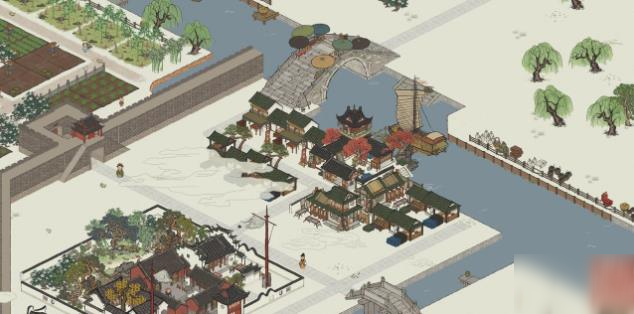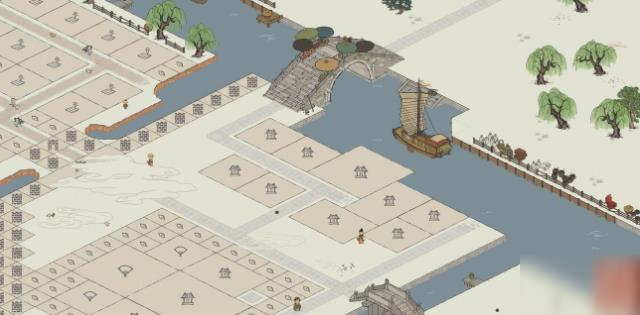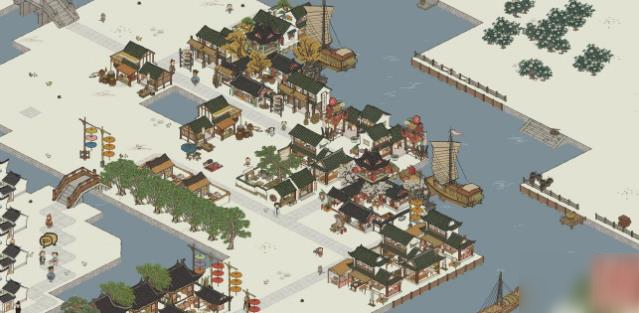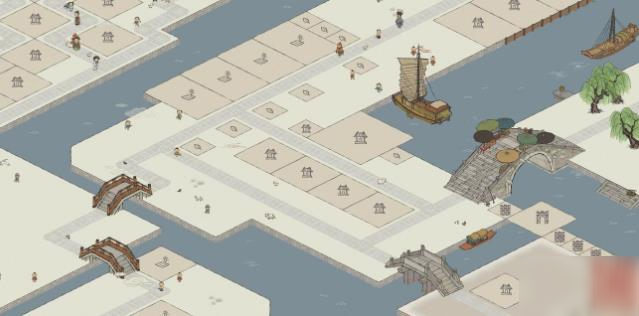33300/100=333×78=25974 百分之七十八加成

33300/100=333×150=49950 天赋百分之一百五加成

33300+25974+49950=109224333×50=16650 天赋百分之五十加成

33300+25974+16650=75924400000/265=1509.433962/12小时=125.7861635。